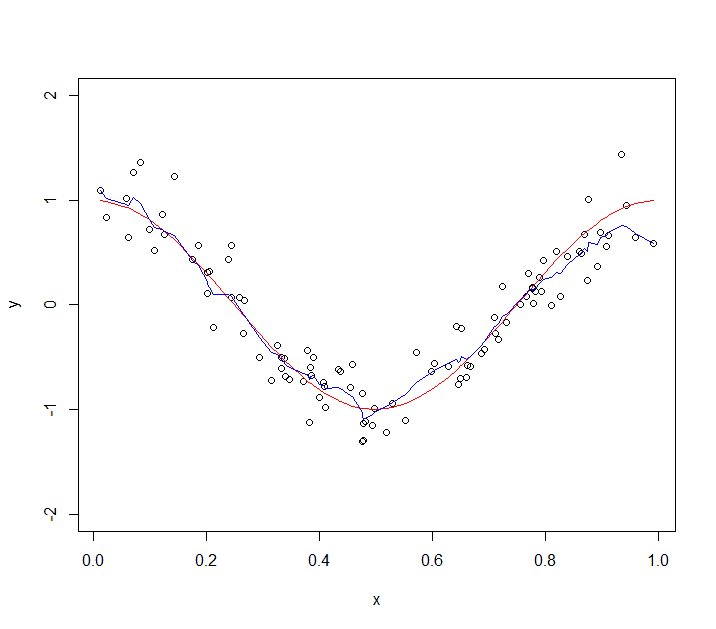## Tuesday, August 20, 2013

### Weak Learners

Tonight's session of R bore just enough fruit that I finally am writing my sophomore entry. I was browsing the slides from a machine learning lecture given by Leo Breiman and came across a relatively simple example he used to introduce the notions of weak and strong learners en route to bagging.

Suppose we are interested in approximating a function $f(x)$ based on observed data $(x_1, y_1), \ldots, (x_n,y_n)$. Given some error in measurement due to outside influence or physical limitations, we'd like to find some method to approximate $f(x)$.

From the set of observed data, resample $m$ points in the interior. Taking these points along with the boundary values gives us a total of $m+2$ coordinate pairs. We now connect consecutive points $(x_i,y_i)$ and $(x_{i+1},y_{i+1})$ by calculating the unique line through that pair. We restrict each such line's domain to values between $x_i$ and $x_{i+1}$. This process will ultimately construct a piecewise function of the form:
$$WL(x) = \sum_{i=1}^{m+1} \left[ \left( \frac{y_{i+1}-y_i}{x_{i+1}-x_i} \right) (x-x_i)+y_i \right] \times I_{[x_i,x_{i+1}]}(x)$$
Where $I_{[x_i,x_{i+1}]}(x)$ is the indicator function, equal to 1 on the designated set and 0 elsewhere.Each line segment connects a pair of consecutive points from our random subset.

The above piecewise function will be called a weak learner. We can generate $k-1$ further weak learners by resampling a different $m$ interior points. To smooth the $k$ weak learners, we average their predicted values. My code appears below for you to test, play with, and critique. How does the smooth learner behave as we increase the number of weak learners? Do you notice/expect any restrictions?

(My LaTeX converter caused me some grief, so you'll have to remove the "\" from each "\$" if you want to run my code.) #Weak and Smoothed Learners weak.learner = function(x, y) { n = length(x) m = max(1, ceiling(n/5) ) s = sample(2:(n-1), size = m, replace = FALSE, prob = NULL) s = c(1,sort(s),n) sx = x[s]; sy = y[s] wl = matrix(nrow = (m+1), ncol = 4) for ( i in 1:(m+1)) { c1 = (sy[i+1]-sy[i])/(sx[i+1]-sx[i]) #slope c0 = sy[i] - c1*sx[i] #intercept wl[i,1] = c0; wl[i,2] = c1 wl[i,3] = sx[i]; wl[i,4] = sx[i+1] #end points for line } wl = data.frame(c0 = wl[,1], c1 = wl[,2], a = wl[,3], b = wl[,4]) wl.hat = matrix(nrow = n, ncol = 1) for (i in 1:n) { wl.hat[i] = sum((wl\$c0 + wl\$c1*x[i])*ifelse(wl\$a <= x[i] & x[i] < wl\$b, 1, 0)) } wl.hat[n] = sum((wl\$c0 + wl\$c1*x[n])*(ifelse(wl\$a < x[i] & x[i] <= wl\\$b, 1, 0)))
return(wl.hat)
}
smooth.learner = function(x,y,k) {
n = length(x)
learners = matrix(nrow = k, ncol = n)
for (i in 1:k) {learners[i,] = weak.learner(x,y)}
smooth = apply(X = learners, MARGIN = 2, FUN = mean)
return(smooth)
}

# Example Usage:
set.seed(1)
#f = function(x){ifelse(x<.5,0,1)}
f = function(x){cos(2*pi*x)}
n = 100
x = sort(runif(n))
y = f(x) + rnorm(n, mean = 0, sd = .25)
yhat = smooth.learner(x,y,k = 50)
plot(x,y,col="BLACK",ylim=c(-2,2) )
points(x,f(x),col="RED",type="l")
points(x,yhat,col="BLUE",type="l")This example uses a cosine function with normal errors at each observation.

1.Hi David, nice post!

I've been learning about ensemble methods as well. I'm mostly doing classification rather than regression, so it's a slightly different formulation. It's more of a voting process among the weak learners, rather than averaging.

-Brian

2.Thanks, Brian. I have been on a reading kick for interpolation and approximation, so this method jumped out at me. It was meant to serve as an introduction to other methods like bagging and boosting, so classification is just around the corner.

There were a couple errors in my original code and the piecewise formula, so I am fixing those. The original smoothed learner should have been called a spiky learner, since I neglected to include only left-hand endpoints in the indicator functions. The result was that multiple parts of the piecewise function could be "on" at each endpoint, inflating the predicted values.